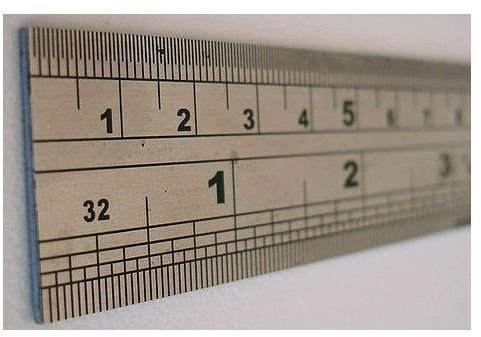# Formulas and Examples of How to Convert Cubic Feet into Cubic Yards

Page content

## Converting Between Different Units of MeasurementWorking with different units of measurement is an important skill for everyone to master. If you are cooking, you need to be able to understand the difference between tablespoons and cups, for example. If you are building a large container, you may want to know how to convert cubic feet into cubic yards. It is also valuable to be able to convert between metric and standard units of measurement. For example, understanding how to convert miles to kilometers is something you need to know if you are visiting a country that uses the metric system like Canada or Europe.

Converting between units of measurement also improves communication. Imagine how difficult the speed limit on highways would be to post on signs if it was communicated as 105,600 yards per hour (or 3,801,600 inches per hours) instead of the easy to read “60 miles per hour.” Knowing which unit of measurement to use in different situations takes time and experience to learn. Learning how to convert between different measurements will make you more flexible and able to work with a wide variety of numbers.

## Formula and Examples

If you are working on a homework assignment with questions on unit conversion, you can use the formulas and examples in this article to work through the problems. In more complex problems, you may be required to convert between multiple sets of units. It is very easy to get confused when converting between different units of measurement, so clearly indicate which unit you are dealing with as you come up with an answer.

To get started with learning how to convert cubic feet into cubic yards, use this simple rule: there are twenty seven (27) cubic feet in one (1) cubic yard. That means that 54 cubic feet would convert to two cubic yards. On the other hand, an object with a volume of one hundred cubic yards would be equivalent to 2700 cubic feet. You can use this article to make both kinds of conversions: cubic feet to cubic yards and cubic yards to cubic feet.

Cubic Yards Into Cubic Feet Examples

• 1 cubic yard is equivalent to 27 cubic feet
• 5 cubic yards are equivalent to 135cubic feet
• 10 cubic yards are equivalent to 270 cubic feet
• 50 cubic yards are equivalent to 1350 cubic feet
• 100 cubic yards are equivalent to 2700 cubic feet
• 1000 cubic yards are equivalent to 27,000 cubic feet

Cubic Feet Into Cubic Yards Examples

• 1 cubic foot is equivalent to 0.037 cubic yards
• 5 cubic feet are equivalent to 0.185 cubic yards
• 10 cubic feet are equivalent to 0.37 cubic yards
• 50 cubic feet are equivalent to 1.85 cubic yards
• 100 cubic feet are equivalent to 3.7 cubic yards
• 1000 cubic feet are equivalent to 37.03 cubic yards

## Resources

To learn more about converting between units of measurement and how to convert cubic feet into cubic yards, consider the resources listed below.